Question

# A grasshopper jumps a horizontal distance of 1.40 m from rest, with an initial velocity at...

A grasshopper jumps a horizontal distance of 1.40 m from rest, with an initial velocity at a 42.0° angle with respect to the horizontal.

(a) Find the initial speed of the grasshopper.
m/s

(b) Find the maximum height reached.
m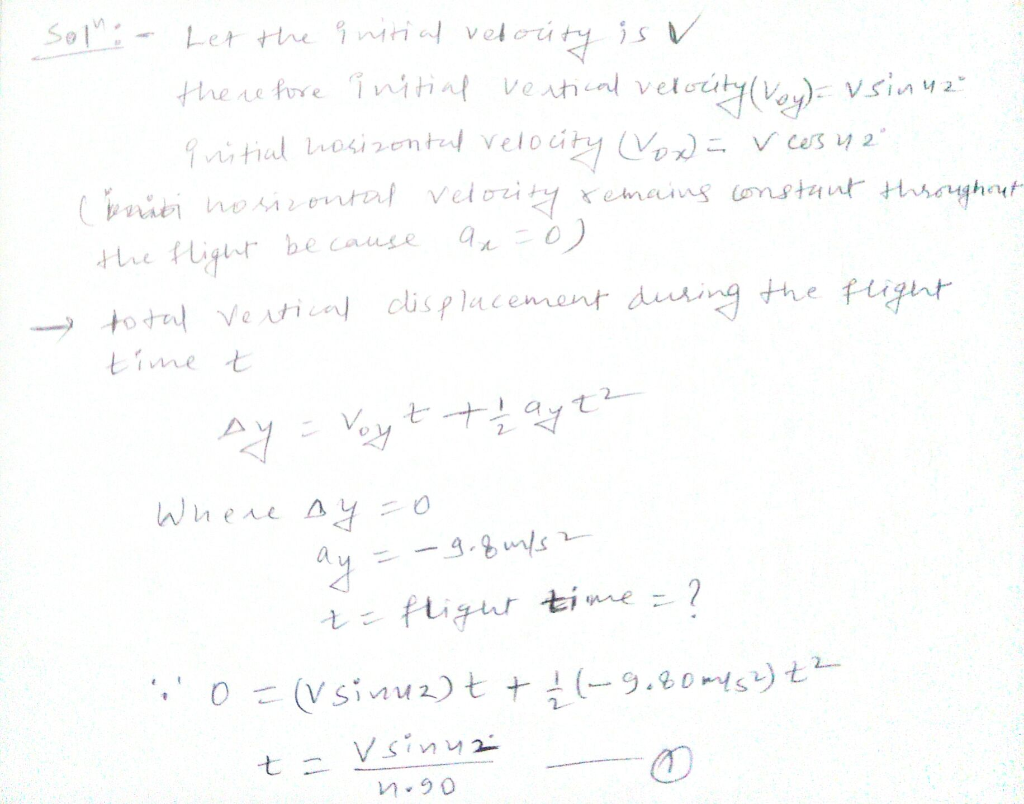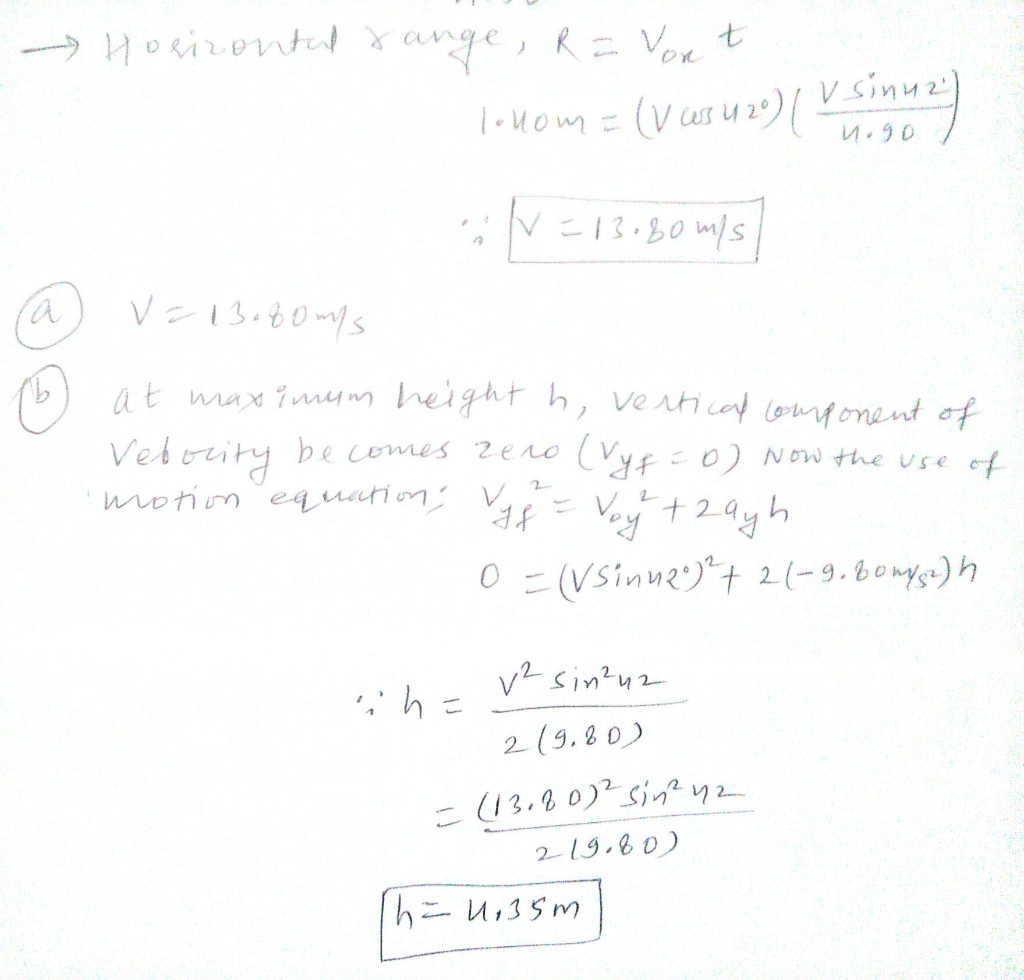#### Earn Coins

Coins can be redeemed for fabulous gifts.

Similar Homework Help Questions
• ### A grasshopper jumps a horizontal distance of 1.40 m from rest, with an initial velocity at...

A grasshopper jumps a horizontal distance of 1.40 m from rest, with an initial velocity at a 50.0° angle with respect to the horizontal. (a) Find the initial speed of the grasshopper. m/s (b) Find the maximum height reached. m

• ### A grasshopper jumps a horizontal distance of 1.50 m from rest, with an initial velocity at...

A grasshopper jumps a horizontal distance of 1.50 m from rest, with an initial velocity at a 40.0° angle with respect to the horizontal. (a) Find the initial speed of the grasshopper. m/s (b) Find the maximum height reached. m

• ### Projectile motion (exercise 3.6)

I need help solving the following question:A grasshopper jumps a horizontal distance of 1.00 m from rest,with an initial velocity at 45.0 degree angle with respect to thehorizontal.Find (a) theinitial speed of the grasshopper and(b) the maximum height reached.

• ### A soccer ball is kicked with an initial horizontal velocity of 18 m/s and an initial...

A soccer ball is kicked with an initial horizontal velocity of 18 m/s and an initial vertical velocity of 13 m/s. 1) What is the initial speed of the ball? 2) What is the initial angle ? of the ball with respect to the ground? 3) What is the maximum height the ball goes above the ground? 4) How far from where it was kicked will the ball land? 5) What is the speed of the ball 0.8 seconds after...

• ### A soccer ball is kicked with an initial horizontal velocity of 20 m/s and an initial vertical velocity of 16 m/s

A soccer ball is kicked with an initial horizontal velocity of 20 m/s and an initial vertical velocity of 16 m/s. I've calculated: Initial Speed: 25.61 m/s Angle: 38.66 degrees Maximum Height it reaches: 13.06 m Distance kicked: 65.29m NOW I NEED: What is the speed of the ball 0.7 seconds after it was kicked? How high above the ground is the ball 0.7 seconds after it is kicked? help? please.

• ### A ball is thrown from a height of 20 meters with an initial velocity of 10...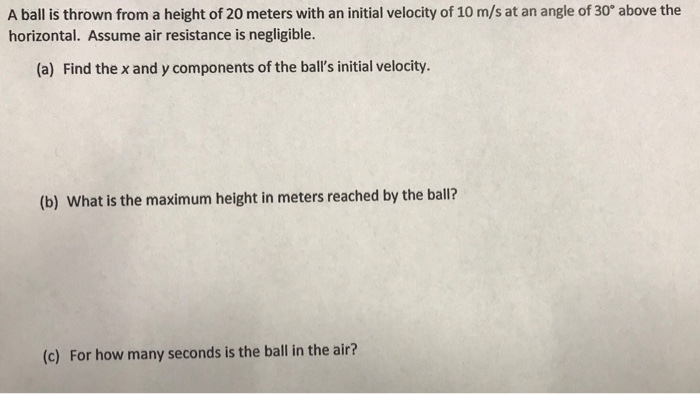A ball is thrown from a height of 20 meters with an initial velocity of 10 m/s at an angle of 30° above the horizontal. Assume air resistance is negligible. (a) Find the x and y components of the ball's initial velocity. (b) What is the maximum height in meters reached by the ball? (c) For how many seconds is the ball in the air?

• ### ASSIGNMENT PROBLEMS 1: A projectile is fired with an initial speed of 60.0 m/s at an...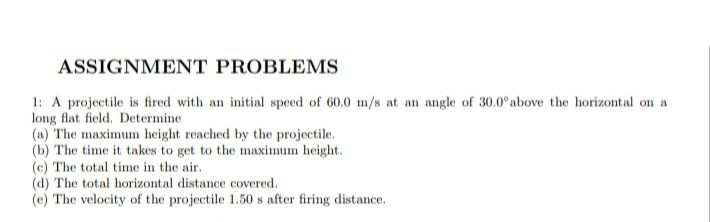ASSIGNMENT PROBLEMS 1: A projectile is fired with an initial speed of 60.0 m/s at an angle of 30.0°above the horizontal on a long flat field. Determine (a) The maximum height reached by the projectile. (b) The time it takes to get to the maximum height. (c) The total time in the air. (d) The total horizontal distance covered. (e) The velocity of the projectile 1.50 s after firing distance.

• ### In the figure, a stone is projected at a diff of height h with an initial...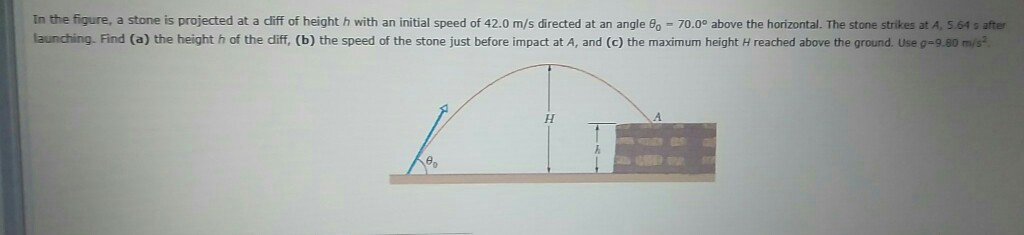In the figure, a stone is projected at a diff of height h with an initial speed of 42.0 m/s directed at an angle θο-70.0 above the horizontal. The stone strikes at A, S 61 s after launching. Find (a) the height h of the diff, (b) the speed of the stone just before impact at A, and (c) the maximum height H reached above the ground. Use 9-9.80 m/s

• ### What is the horizontal distance covered by a thrown ball if it has an initial velocity...

What is the horizontal distance covered by a thrown ball if it has an initial velocity of 18 m/s at an angle of 37 degrees relative to horizontal with an initial height of release of 2 meters?

• ### An object is launched with an angle of 25 upward with the horizontal. The initial velocity...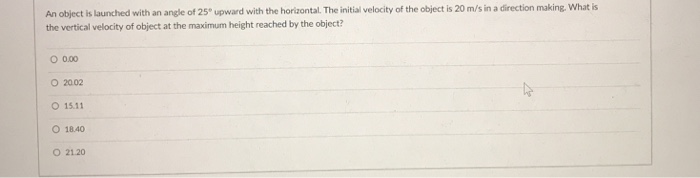An object is launched with an angle of 25 upward with the horizontal. The initial velocity of the object is 20 m/'s in a direction making. What is the vertical velocity of object at the maximum height reached by the object? 0.00 O 15.11 O 18.40 O 21.20